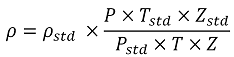# Stream Density Calculation (based on Standard Density)

### Stream Density Calculation (based on Standard Density)

#### This method is used to calculate the stream density from the standard density. For flow meters other than differential pressure flow meters the pressure and temperature at the flow meter is entered in the place of upstream pressure and temperature.

Upstream density is calculated as follows:Symbol Description Units
ρ Density at the upstream conditions kg/m3
ρstd Standard density kg/m3
P Pressure at the upstream conditions bar a
Pstd Standard pressure bar a
T Temperature at the upstream conditions K
Tstd Standard temperature K
Z Compression factor at the upstream conditions -
Zstd Compression factor at standard conditions -